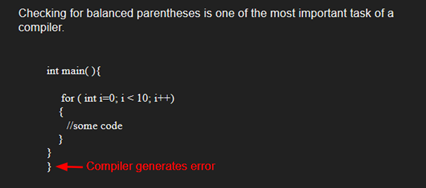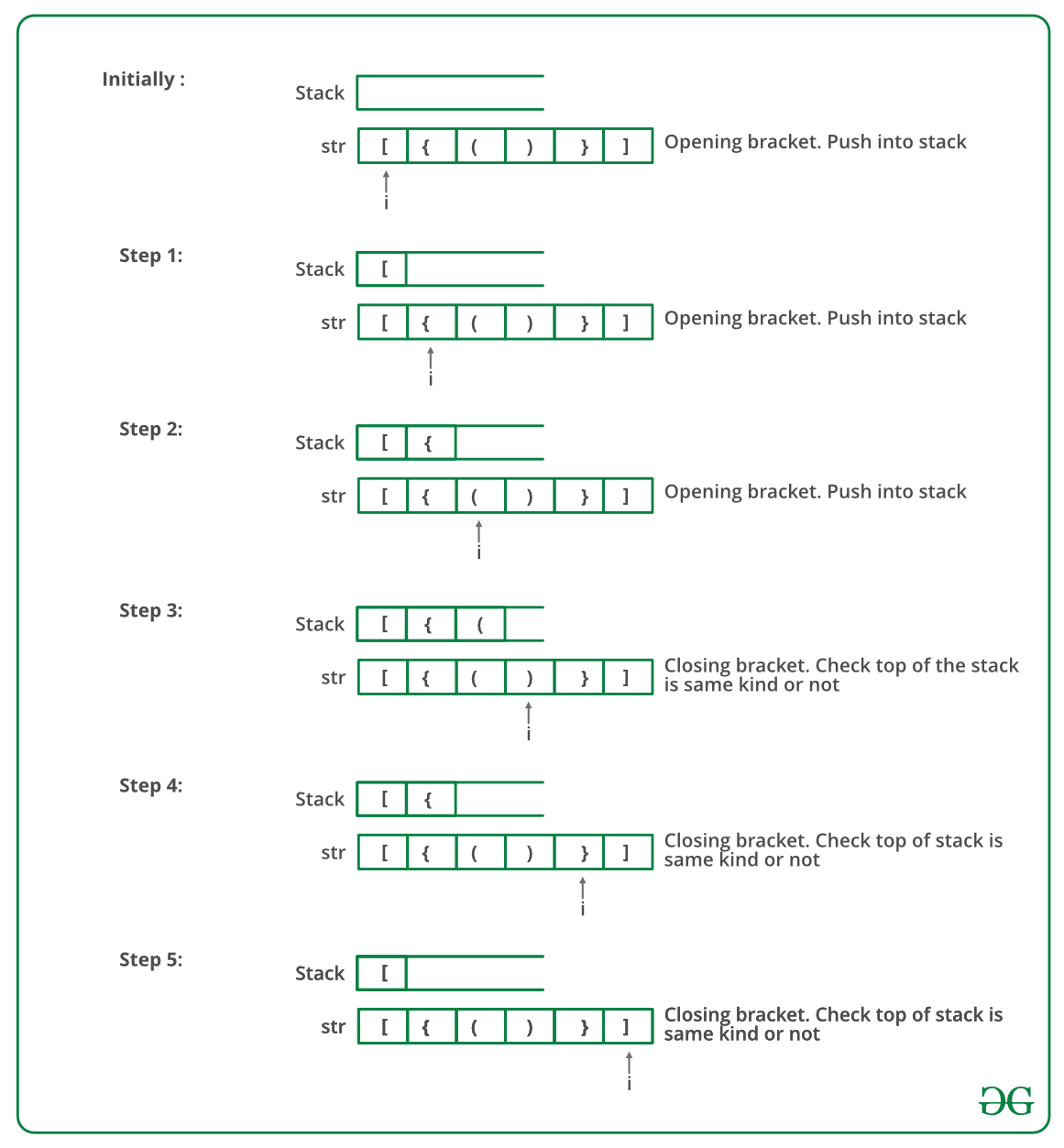# C# Program To Check For Balanced Brackets In An Expression (Well-Formedness) Using Stack

• Last Updated : 15 Dec, 2021

Given an expression string exp, write a program to examine whether the pairs and the orders of “{“, “}”, “(“, “)”, “[“, “]” are correct in exp.

Example

Input: exp = “[()]{}{[()()]()}”
Output: Balanced

Input: exp = “[(])”
Output: Not BalancedAlgorithm:

• Declare a character stack S.
• Now traverse the expression string exp.
1. If the current character is a starting bracket (‘(‘ or ‘{‘ or ‘[‘) then push it to stack.
2. If the current character is a closing bracket (‘)’ or ‘}’ or ‘]’) then pop from stack and if the popped character is the matching starting bracket then fine else brackets are not balanced.
• After complete traversal, if there is some starting bracket left in stack then “not balanced”

Below image is a dry run of the above approach:Below is the implementation of the above approach:

## C#

 `// C# program for checking``// balanced Brackets``using` `System;``using` `System.Collections.Generic;`` ` `public` `class` `BalancedBrackets {``    ``public` `class` `stack {``        ``public` `int` `top = -1;``        ``public` `char``[] items = ``new` `char``;`` ` `        ``public` `void` `push(``char` `x)``        ``{``            ``if` `(top == 99) ``            ``{``                ``Console.WriteLine(``"Stack full"``);``            ``}``            ``else` `{``                ``items[++top] = x;``            ``}``        ``}`` ` `        ``char` `pop()``        ``{``            ``if` `(top == -1) ``            ``{``                ``Console.WriteLine(``"Underflow error"``);``                ``return` `'�'``;``            ``}``            ``else` `            ``{``                ``char` `element = items[top];``                ``top--;``                ``return` `element;``            ``}``        ``}`` ` `        ``Boolean isEmpty()``        ``{``            ``return` `(top == -1) ? ``true` `: ``false``;``        ``}``    ``}`` ` `    ``// Returns true if character1 and character2``    ``// are matching left and right brackets */``    ``static` `Boolean isMatchingPair(``char` `character1,``                                  ``char` `character2)``    ``{``        ``if` `(character1 == ``'('` `&& character2 == ``')'``)``            ``return` `true``;``        ``else` `if` `(character1 == ``'{'` `&& character2 == ``'}'``)``            ``return` `true``;``        ``else` `if` `(character1 == ``'['` `&& character2 == ``']'``)``            ``return` `true``;``        ``else``            ``return` `false``;``    ``}`` ` `    ``// Return true if expression has balanced``    ``// Brackets``    ``static` `Boolean areBracketsBalanced(``char``[] exp)``    ``{``        ``// Declare an empty character stack */``        ``Stack<``char``> st = ``new` `Stack<``char``>();`` ` `        ``// Traverse the given expression to``        ``//   check matching brackets``        ``for` `(``int` `i = 0; i < exp.Length; i++) ``        ``{``            ``// If the exp[i] is a starting``            ``// bracket then push it``            ``if` `(exp[i] == ``'{'` `|| exp[i] == ``'('``                ``|| exp[i] == ``'['``)``                ``st.Push(exp[i]);`` ` `            ``//  If exp[i] is an ending bracket``            ``//  then pop from stack and check if the``            ``//   popped bracket is a matching pair``            ``if` `(exp[i] == ``'}'` `|| exp[i] == ``')'``                ``|| exp[i] == ``']'``) {`` ` `                ``// If we see an ending bracket without``                ``//   a pair then return false``                ``if` `(st.Count == 0) ``                ``{``                    ``return` `false``;``                ``}`` ` `                ``// Pop the top element from stack, if``                ``// it is not a pair brackets of``                ``// character then there is a mismatch. This``                ``// happens for expressions like {(})``                ``else` `if` `(!isMatchingPair(st.Pop(),``                                         ``exp[i])) {``                    ``return` `false``;``                ``}``            ``}``        ``}`` ` `        ``// If there is something left in expression``        ``// then there is a starting bracket without``        ``// a closing bracket`` ` `        ``if` `(st.Count == 0)``            ``return` `true``; ``// balanced``        ``else` `        ``{ ``            ``// not balanced``            ``return` `false``;``        ``}``    ``}`` ` `    ``// Driver code``    ``public` `static` `void` `Main(String[] args)``    ``{``        ``char``[] exp = { ``'{'``, ``'('``, ``')'``, ``'}'``, ``'['``, ``']'` `};`` ` `        ``// Function call``        ``if` `(areBracketsBalanced(exp))``            ``Console.WriteLine(``"Balanced "``);``        ``else``            ``Console.WriteLine(``"Not Balanced "``);``    ``}``}`` ` `// This code is contributed by 29AjayKumar`
Output
`Balanced`

Time Complexity: O(n)
Auxiliary Space: O(n) for stack.

Please refer complete article on Check for Balanced Brackets in an expression (well-formedness) using Stack for more details!

My Personal Notes arrow_drop_up